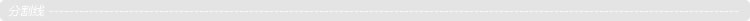# 从零开始，DIY一个jQuery（3）

    jQuery.fn.init = function (selector, context, root) {
if (!selector) {
return this;
} else {
var elem = document.querySelector(selector);
if (elem) {
this = elem;
this.length = 1;
}
return this;
}
};

$('div').find('p').find('span') 很明显，这可以在 jQuery.fn.extend 里多加一个 find 接口来实现，不过不着急，咱们一步一步来。2.$.merge 方法

jQuery.fn = jQuery.prototype = {
jquery: version,
length: 0,  // 修改点1，JQ实例.length 默认为0
//...
}

jQuery.extend( {
merge: function( first, second ) {  //修改点2，新增 merge 工具接口
var len = +second.length,
j = 0,
i = first.length;

for ( ; j < len; j++ ) {
first[ i++ ] = second[ j ];
}

first.length = i;

return first;
},
//...
});

merge 的代码段太好理解了，其实现的能力为：

<div>hello</div>
<div>world</div>

<script>
var divs = $.find('div'); //纯数组 var$div1 = $.merge( ['hi'], divs); //右边的数组合并到左边的数组，形成一个新数组 var$div2 = $.merge( {0: 'hi', length: 1}, divs); //右边的数组合并到左边的对象，形成一个新的类数组对象 console.log($div1);
console.log($div2); </script> 运行输出：因此，如果我们在 jQuery.fn.init 中，把 this 传入为$.merge 的 first 参数（留意这里this为JQ实例对象自身，默认 length 实例属性为0），再把检索到的 DOM 集合数组作为 second 参数传入，那么就能愉快地得到我们想要的 JQ 类数组对象了。

    jQuery.fn.init = function (selector, context, root) {
if (!selector) {
return this;
} else {
var elemList = jQuery.find(selector);
if (elemList.length) {
jQuery.merge( this, elemList );  //this是JQ实例，默认实例属性 .length 为0
}
return this;
}
};

<div>hello</div>
<div>world</div>

<script>
var $div =$('div');
console.log($div); </script> 输出正是我们所想要的类数组对象：3. 扩展$.fn.find

</script>jQuery.fn.extend({
find: function( selector ) {  //链式支持find
var i, ret,
len = this.length,
self = this;

ret = [];

for ( i = 0; i < len; i++ ) {  //遍历
jQuery.find( selector, self[ i ], ret );  //直接利用 Sizzle 接口，把结果注入到 ret 数组中去
}

return this.pushStack( ret );  //转为JQ对象
}
});

jQuery.fn.extend({
find: function( selector ) {  //链式支持find
var i, ret,
len = this.length,
self = this;

ret = this.pushStack( [] ); //转为JQ对象

for ( i = 0; i < len; i++ ) {  //遍历
jQuery.find( selector, self[ i ], ret );  //直接利用 Sizzle 接口，把结果注入到 ret 数组中去
}

return ret
}
});4. $.fn.end、$.fn.eq 和 $.fn.get 鉴于我们在 pushStack 中加上了 oldJQ.prevObject 的关系链，那么$.fn.end 接口的实现就太简单了：

jQuery.fn.extend({
end: function() {
return this.prevObject || this.constructor();
}
});

jQuery.fn.extend({
end: function() {
return this.prevObject || this.constructor();
},
eq: function( i ) {
var len = this.length,
j = +i + ( i < 0 ? len : 0 );  //支持倒序搜索，i可以是负数
return this.pushStack( j >= 0 && j < len ? [ this[ j ] ] : [] ); //容错处理，若i过大或过小，返回空数组
},
get: function( num ) {
return num != null ?

// 支持倒序搜索，num可以是负数
( num < 0 ? this[ num + this.length ] : this[ num ] ) :

// 克隆一个新数组，避免指向相同
[].slice.call( this );  //建议把 [].slice 封装到 var.js 中去复用
}
});

jQuery.fn.extend({
first: function() {
return this.eq( 0 );
},
last: function() {
return this.eq( -1 );
}
});2. 同上，$.fn.find 也未对兼顾到各种参数类型的情况； 3.$.fn.find 返回结果有可能带有重复的 DOM，例如：

<div><div><span>hi</span></div></div>

<script>
var $span =$('div').find('span');
console.log(\$span);  //重复了
</script>

1. 部分读者是从公众号上阅读本系列文章的，建议也要同时关注本人博客好一些 —— 有时我会对文章做一些更改，让其更易读懂；
2. 对于前两篇文章，部分基础较差的读者貌似不太好理解，我其实有考虑写个番外篇来帮你们梳理这块（特别是原型链的）知识点，如果觉得有需要的话可以留言给我，要求的人多的话我就动笔了；
3. 工作较忙，发文频率大约是1到2周一篇文章。近期其实蛮多读者催我更文的，但为了保持文章质量，需要多点时间，不希望数量上来了质量却下去了。posted @ 2016-08-15 01:44  vajoy  阅读(2623)  评论(8编辑  收藏  举报